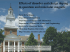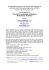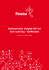Phase Effects in the Nonlinear Interaction of “Negative”

Transcription

Phase Effects in the Nonlinear Interaction of “Negative”
```206
F. ENGELMANN AND H. WILHELMSSON
Phase Effects in the Nonlinear Interaction of “Negative”-Energy Waves
F. E n g e l m a n n and H. W
il h e l m s s o n
*
Laboratori Gas Ionizzati (Associazione EURATOM -CNEN ), Frascati (Rome), Italy
(Z. Naturforsdi. 24 a, 206— 207  ; received 30 September 1968)
Nonlinear interaction of “negative”-energy waves is studied assuming well-defined phases and
perfect matching conditions. The results are compared with those of the random-phase approach,
and significant differences in the dynamics due to phase effects are pointed out.
The lowest-order nonlinear interaction of three
monochromatic waves, with well-defined phases, hav­
ing the frequencies co0 , « j , 0 ) 2 where
oj0= oj1 + o) 2
,
(2 a)
3 r..,
sign |fg-—
[ o j j 2 e { a ) j)
(2 b)
si ui~ ~ s 2 u 2 - m 12 ,
du2/d r=
s0 s1 u 0 u 1 cos 0 ,
(2 c)
50
0
sin 0 .
S0 S2
dr
]
(5)
From Eqs. (2) and (3) one obtains
,
d (» o 2)
all Sj are equal, or
5X52= —1 and s0 = arbitrary;
(6 b)
S0 U02 + S1 u 2 = m 0 1 ,
(6 c)
-)-
S2 U 22 =
//Iq o
u 0 ux u 2 sin & = T
(7)
with the m’s and r integration constants, and
(8)
of the waves has negative energy (in any frame of
reference) 4-6. Qualitatively, the evolution of the
Uj2 and 0 is readily obtained from the preceding
relations (cf. Fig. 1). For U j2
oo one has
51ä2= +1 and 50 = —5 != —s2
(or, equivalently, 5„ Sl = s„ , s = - 1).
(6 a)
,
U q"
— —2 l/ ^ 52[u0" (m01 —50 u02) (m 02 —s0 u02) —7"2] sign[cos 0].
Two different cases may occur:
II)
=
s0 s2 u0 u2 cos
\$1 «So
1)
2)
Sj
th amplitude, and
d u jd x =
(3)
I)
j the phase of the
with
(4)
e(toj) being the dielectric constants.
du0/dr = —Sj s2 ux u 2 cos
dr
2> = <P0
(1)
can be shown to be described by
d<P
The Uj > 0 are the moduli of the wave amplitudes,
^
^
Uj2^ (too - r) ~ 2
(9)
depending on ^
conditions Hence>
Case I has been extensively treated 1-3 with the resuit that the U j ( r ) are oscillatory and bounded.
Physically, it corresponds to an interaction of waves
of positive energy (in an appropriate frame of re­
ference) .
We are interested here in case II, for which all
duj/dr have the same sign. Hence, all amplitudes
vary in the same sense, which is only possible if one
the increase of the wave amplitudes — after a transient period, depending on |
| and during which
the uj may even decrease — is faster than exponen­
tial ' and leads to a divergence at a finite t x . At the
same time, the phase is locked 7 to 0 = ± n for sign
^ o = — 1’ irrespective of
• W e note that, as in
case I, one can solve Eqs. (2) and (3) exactly in
terms of elliptic functions 1’ 3.
* Permanent address: Institute of Physics, University of Uppsala, Sweden.
1 J. A. A r m s t r o n g , N. B l o e m b e r g e n , J. D u c u i n g , and P. S.
P e r s h a n . P h y s . Rev. 127, 1918 .
2 A. S j ö l u n d and L. S t e n f l o , Appl. Phys. Lett. 10, 201
 ; Physica 33, 499 .
3 R. S u g i h a r a , Phys. Fluids 11, 178 .
4 B. B.
K a d o m t s e v , A. B. M i k h a i l o v s k i i , and A. V. T i m o Sov. Phys. JE T P 20, 1517 .
5 V. M. D i k a s o v , L. I. R u d a k o v , and D . D . R y u t o v , S o v .
Phys. JETP 21. 608 .
0 M. N. R o s e n b l u t h , B. C o p p i , and R. N. S u d a n , 3 rd Conf.
on Plasma Phys. and Contr. Nucl. Fusion Res. Novosibirsk
1968. Paper CN 24/E13.
7 Cf. also P. A. S t u r r o c k , Phys. Rev. Lett. 16. 270 .
feev,
Dieses Werk wurde im Jahr 2013 vom Verlag Zeitschrift für Naturforschung
in Zusammenarbeit mit der Max-Planck-Gesellschaft zur Förderung der
Wissenschaften e.V. digitalisiert und unter folgender Lizenz veröffentlicht:
Creative Commons Namensnennung-Keine Bearbeitung 3.0 Deutschland
Lizenz.
This work has been digitalized and published in 2013 by Verlag Zeitschrift
für Naturforschung in cooperation with the Max Planck Society for the
Zum 01.01.2015 ist eine Anpassung der Lizenzbedingungen (Entfall der
Creative Commons Lizenzbedingung „Keine Bearbeitung“) beabsichtigt,
um eine Nachnutzung auch im Rahmen zukünftiger wissenschaftlicher
Nutzungsformen zu ermöglichen.
On 01.01.2015 it is planned to change the License Conditions (the removal
of the Creative Commons License condition “no derivative works”). This is
to allow reuse in the area of future scientific usage.
NONLINEAR INTERACTION OF NEGATIVE-ENERGY WAVES
207
Let us consider the same interaction problem in
random-phase approximation. The corresponding
equations of motion are 5’ 6>8’ 9
d K 2)
—
- 2 —2
= Ux~ U
-
—2 —2
—0 —2
s0 Sx u 0z U .S - s0 So u 0~ u x%
[1f.\
(10)
and two similar equations for d(üx2)/df and d(ü22)/df,
from which the validity of Eqs. (6) in terms of the
üj2 is easily shown. By using them, exact solutions
for the üj2(f) are readily obtained. For üj2—> oo ,
one has in case II
üj2^ \ { r o o - t)“ 1
(11)
with rx determined by the initial conditions. Hence,
the final increase of the wave amplitudes is less “ex­
plosive” 7 here than in the case of determined phase.
On the other hand, no decrease of the amplitudes,
even transitory, is possible in the random-phase
case.
In conclusion, phase effects may have consider­
able importance for the dynamics of the considered
wave interaction.
Fig. 1. Qualitative plot of a) u0z as a function of r with the
modulus |0 O|as a parameter, assuming the initial value u002
of u02 larger than either u102 or u202, and b) 0 as a function
of u02; the arrows refer to increasing r.
H.
W i l h e l m s s o n wishes to thank Prof. B. B r u n e l l i
for the kind hospitality extended to him at the Laboratori Gas Ionizzati at Frascati.
8 V. N.
9 R. E. A a m o d t and M. L.
.
T s y t o v ic h , Sov.
Phys. Uspekhi 9, 805 .
S lo a n ,
Phys. Rev. Lett. 19, 1227
```

Strategies for Exploring the Human Side of Change â Presenter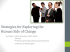PHYSICS 51, Sec 5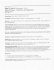Flipping Intro Physics at the University of Illinois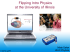Exam 2B - KEY - faculty.piercecollege.edu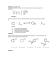Everyone should have a clicker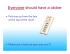WWW hhh aaa ttt iii sss PPP hhh yyy sss iii ccc sss AAA ppp ppp lll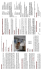Electron Paramagnetic Resonance of Ni2+ in BaZnF4 Single Crystals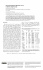EE, MATH & ME Admissions Planning Worksheet 2014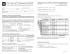Exact exponential solutions in Einstein-Gauss - Quarks-2014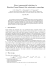Percolation noise at the metalâinsulator transition of nanostructured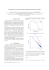M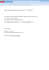Talk Abstracts May 21 - CIQM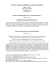megawave - James Law Cybertecture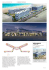1.68MB - Waves Inc.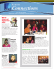Poster - Department of Physics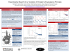Slides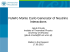Stars and Planets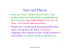I Br Cl CN SH SR N C CR OH OR NH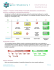100 lat fizyki niskich temperatur i nadprzewodnictwa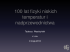Effects of disorder and charge doping in quantum and